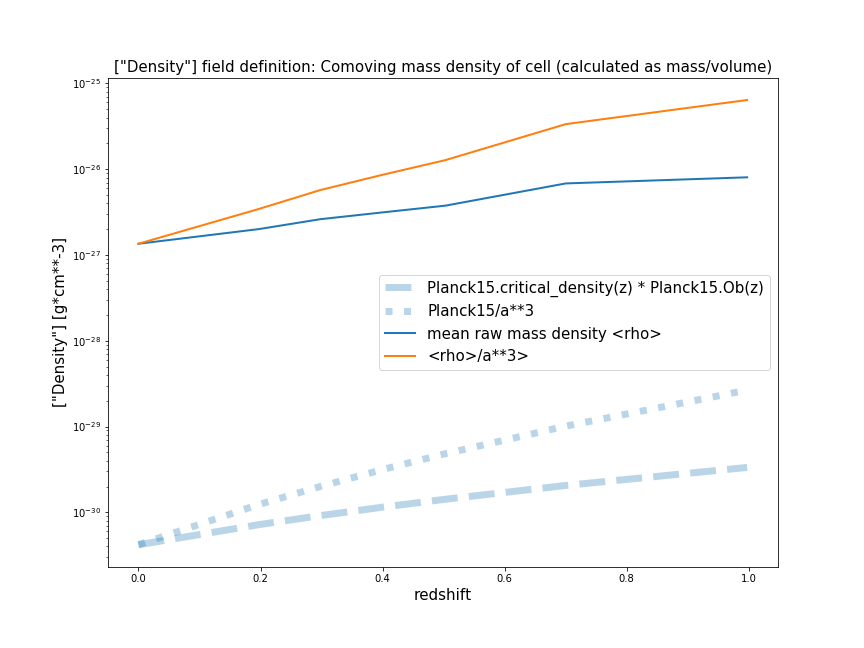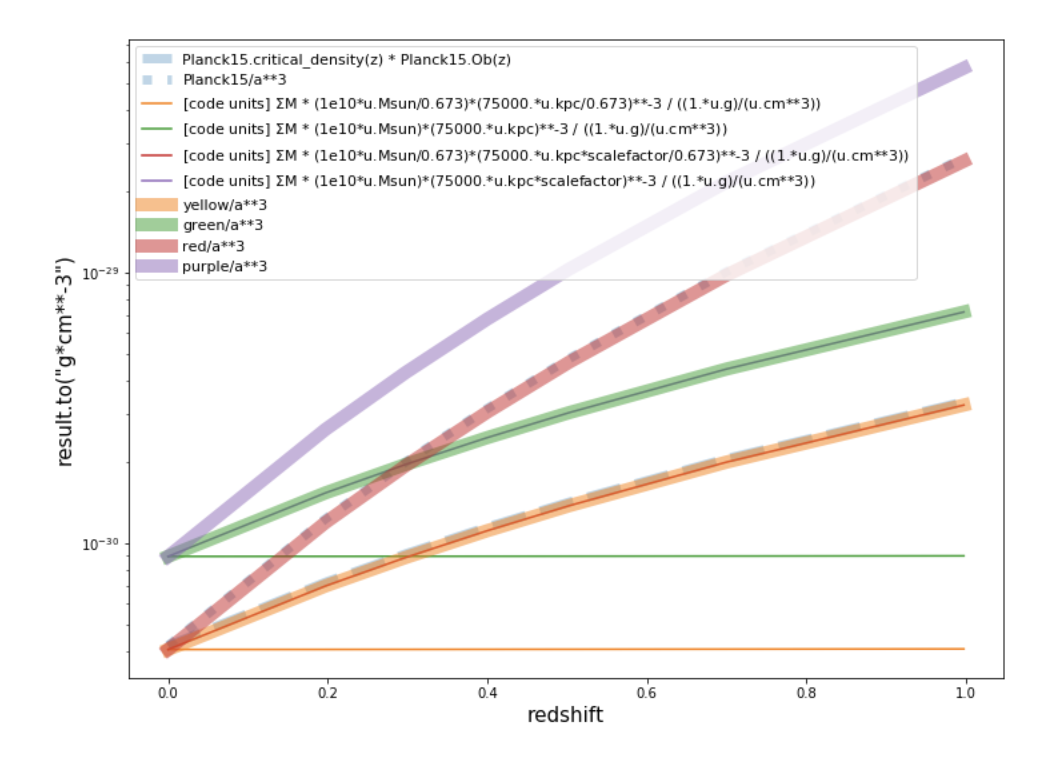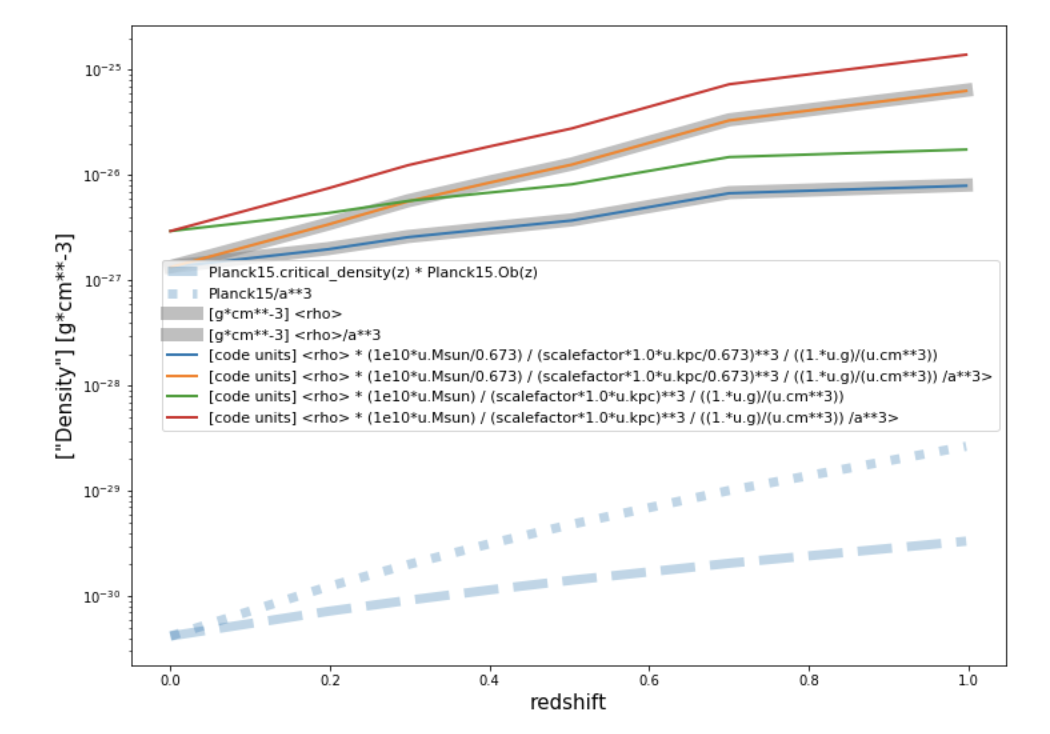### Comparison of average density to theoryCharles Walker
• 13 Sep '21

Hello Dylan, hope you're doing well.

I'm currently investigating an apparent slight underdensity in my work, and I've done a test which confuses me lightly. Please could you. help me clear it up?

I have plotted in dotted llines the theoretical evolution of (critical density * omega baryon) as a function of redshift according to Planck15 results. I used astropy to get these numbers (more specifically, Planck15.Ob() and Planck15.critical_density()).

I have also gone through every main illustris snapshot, extracted the PartType0 (gas) density for each cell, and plotted the mean of these vs redshift.

You can see the pot below, where everything is in units of g/cm**3, and also when shifted by the scale factor.

I would have thought these would be the same (or if anything, the TNG values would be lower as PartType0 only includes gas, not stars). However the TNG values are ~3000 times larger than the theory.

Have I missed something obvious here?

Cheers,

CharlieDylan Nelson
• 13 Sep '21

As I check I would load the `Masses` of all gas cells (instead of Density), and then divide by the volume of the simulation box, to derive the mean gas density.Charles Walker
• 17 Sep '21

Hi Dylan,

Thanks for the suggestion. I've done as you said, and attach the findings below.
The first plot does as you ask: it sums the masses in each cell, and then divides by the size of the snapshot. I did this in code units and figured out the correct conversion factor (i.e. whether to include scale factors and h) myself, and it seems that the answer is 'yes to both' as it is the red lines (thin and thick) which match the theory.
The second plot shows the average of densities as in my original post, but this time I take the code units and transform them using the same method (scale factors and h) as I do in plot 1 of this post. What can be seen is that the output of this matches the plot in my original post (which was an automatic conversion using trident/yt).

So the two methods (averaging the densities and summing the masses) do not yield the same results. I guess this has some mathematical explanation?Dylan Nelson
• 18 Sep '21

This should just be the fact that `(a+b)/(d+e) != a/d + b/e` in general.

So the correct calculation is `rho = sum(mass) / volume_box = (m1+m2+m3+...) / volume_box = (m1+m2+m3+...) / (v1+v2+v3+...)` and although each cell has its own `rho1 = m1/v1`, you cannot make the average `m1/v1 + m2/v2 + ...`. This is only true if all cell volumes are the same, which isn't the case. Another way of saying: you can compute the average density from the cell densities if you weight by cell volume.Charles Walker
• 20 Sep '21

Hi Dylan,

Thanks very much for this. I couldn't for the life of me figure it out, but of course, it was to do with the individual cells being different sizes.

So in other words, I interpret this as meaning, to get average density using the "density" field, you will have to weight each by cell volume, i.e.:

`rho = (dens1)*(v1/vbox) + (dens2)*(v2/vbox) + ...`

But as TNG doesn't provde a "volume" field, this will have to be calculated from density and mass, i.e. `volume=mass/density` and so the above becomes:

`rho = (dens1)*(mass1/dens1)/(vbox) + (dens2)*(mass2/dens2)/(vbox) + ...`

Which simplifies to:

`rho = (mass1)/(vbox) + (mass2/vbox) + ...`

Which is simply the first of my plots in the last post, so in the end, you are not really using the density field in the calculation at all. Correct?

Cheers again,

Charlie

• Page 1 of 1